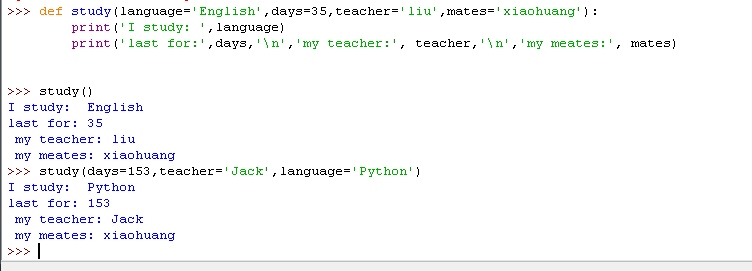• 如果你有一些具有许多参数的函数，而你又希望只对其中的一些进行指定，那么你可以通过命名它们来给这些参数赋值——这就是Python关键字参数——我们使用命名（关键字）而非位置（一直以来我们所使用的方式）来指定...


关键字参数
如果你有一些具有许多参数的函数，而你又希望只对其中的一些进行指定，那么你可以通过命名它们来给这些参数赋值——这就是Python关键字参数——我们使用命名（关键字）而非位置（一直以来我们所使用的方式）来指定函数中的参数。Python中这样做有两大优点——其一，我们不再需要考虑参数的顺序，函数的使用将更加容易。其二，我们可以只对那些我们希望赋予的参数以赋值，只要其它的参数都具有默认参数值。
案例（保存为 function_keyword.py）：
def func(a, b=5, c=10):
print('a is', a, 'and b is', b, 'and c is', c)

func(3, 7)func(25, c=24)func(c=50, a=100)
输出：
$python function_keyword.pya is 3 and b is 7 and c is 10a is 25 and b is 5 and c is 24a is 100 and b is 5 and c is 50 它是如何工作的名为 func 的Python函数有一个没有默认参数值的参数，后跟两个各自带有默认参数值的参数。在第一次调用函数时，func(3, 7)，参数 a 获得了值 3，参数 b 获得了值 7，而 c 获得了默认参数值 10。在第二次调用函数时，func(25, c=24)，由于其所处的位置，变量 a 首先获得了值 25。然后，由于命名——即关键字参数——指定，变量 c 获得了值 24。变量 b 获得默认参数值 5。+ 在第三次调用函数时，func(c=50, a=100)，我们全部使用关键字参数来指定值。在这里要注意到，尽管 a 在 c 之前定义，但我们还是在变量 a 之前指定了变量 c。  展开全文• 如果你有一些具有许多参数的函数，而你又希望只对其中的一些进行指定，那么你可以通过命名它们来给这些参数赋值——这就是Python关键字参数——我们使用命名（关键字）而非位置（一直以来我们所使用的方式）来指定...  关键字参数 如果你有一些具有许多参数的函数，而你又希望只对其中的一些进行指定，那么你可以通过命名它们来给这些参数赋值——这就是Python关键字参数——我们使用命名（关键字）而非位置（一直以来我们所使用的方式）来指定函数中的参数。Python中这样做有两大优点——其一，我们不再需要考虑参数的顺序，函数的使用将更加容易。其二，我们可以只对那些我们希望赋予的参数以赋值，只要其它的参数都具有默认参数值。 案例（保存为 function_keyword.py）： def func(a, b=5, c=10): print('a is', a, 'and b is', b, 'and c is', c)  func(3, 7)func(25, c=24)func(c=50, a=100) 输出：$ python function_keyword.pya is 3 and b is 7 and c is 10a is 25 and b is 5 and c is 24a is 100 and b is 5 and c is 50
它是如何工作的名为 func 的Python函数有一个没有默认参数值的参数，后跟两个各自带有默认参数值的参数。在第一次调用函数时，func(3, 7)，参数 a 获得了值 3，参数 b 获得了值 7，而 c 获得了默认参数值 10。在第二次调用函数时，func(25, c=24)，由于其所处的位置，变量 a 首先获得了值 25。然后，由于命名——即关键字参数——指定，变量 c 获得了值 24。变量 b 获得默认参数值 5。+
在第三次调用函数时，func(c=50, a=100)，我们全部使用关键字参数来指定值。在这里要注意到，尽管 a 在 c 之前定义，但我们还是在变量 a 之前指定了变量 c。


展开全文• def foo(x, y): print(x * x + y) return foo(3, 4) # 3*3+4 = 13 ...#关键字参数 foo(x = 5, y = 9) # 5*5+9 = 34 #关键字参数顺序也可以不按函数声明参数顺序 foo(y = 9, x = 5) # 5*5+9 = 34 ...
def foo(x, y):
print(x * x + y)
return

foo(3, 4)           # 3*3+4  = 13

#关键字参数
foo(x = 5, y = 9)   # 5*5+9  = 34

#关键字参数顺序也可以不按函数声明参数顺序
foo(y = 9, x = 5)   # 5*5+9  = 34

展开全文• 调用使用关键字参数的函数时，以param = value的方式传递数据 好处 ： 1.清晰地指出了参数值，提高程序的可读性； 2.关键字参数不用考虑顺序，对于包含大量参数的函数很有帮助，不用去记住这些函数的参数的顺序和...
调用使用关键字参数的函数时，以param = value的方式传递数据
好处 ：
1.清晰地指出了参数值，提高程序的可读性；
2.关键字参数不用考虑顺序，对于包含大量参数的函数很有帮助，不用去记住这些函数的参数的顺序和含义。转载于:https://www.cnblogs.com/baxianhua/p/10750567.html
展开全文• 关键字参数允许你传入0个或任意个含参数名的参数，这些关键字参数在函数内部自动组装为一个dict。请看示例： 1 #!/usr/bin/env python 2 # -*- coding: utf-8 -*- 3 # 关键字参数:**kw 4 def person(name,age,...
• 关键字参数与非关键字参数（可变参数）详解学过php或者其他语言的同学都知道，php里面的参数不是个数不是可变的（只是很多时候是可以省略的，因为在函数定义的时候为参数设置了默认值）。但是在python里却不是这样，...
• Python关键字参数、位置参数关键字参数位置参数 关键字参数 def fun(a=10,b=20): print(a,b) #关键字参数：根据关键字进行匹配，不需要考虑参数位置的问题 fun(a=1,b=2) fun(b=2,a=1) #默认参数，缺省 fun(1) fun...位置参数
• Python 位置参数、关键字参数、默认参数详解。
• 关键字参数必须跟随在位置参数后面! 因为python函数在解析参数时, 是按照顺序来的, 位置参数是必须先满足, 才能考虑其他可变参数.，否则报错如下：In : print(s1.format(name='jenkins',"good","kg",a='python')...
• 关键字参数 转自https://www.liaoxuefeng.com/wiki/1016959663602400/1017261630425888 记录学习用 可变参数允许你传入0个或任意个参数，这些可变参数在函数调用时自动组装为一个tuple。而关键字参数允许你传入0个...
• This problem originated when I tried to apply a more functional approach to problems in python.What I tried to do is simply square a list of numbers, no biggie.from operator import powfrom functools i...
• 3、关键字参数与参数默认值 1）关键字参数 位置参数：按顺序为每个参数指定参数值 关键字参数（命名参数）：按参数名为参数指定参数值 def info(name, age, height): print('name:', name) print('age:'...参数默认值...

# python关键字参数python 订阅